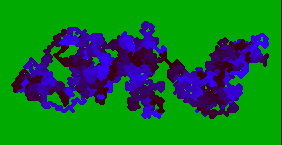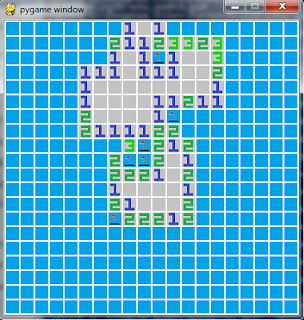## Posts

Showing posts from October, 2017

### Random Walks to solve Diophantine EquationsRandom walks (check my older post here) and Diophantine equations are two simple mathematical beasts. After a college seminar, I tried putting them together to make something neat, and came up with this: just pick a Diophantine equation, simulate a random walk, and try to see if the random walk went over any solutions! In this report I briefly go over how I came up with this, and show the code I wrote/some results. The Matlab code is all in here (I also have some Python code about random walks in general here, check my post!).

In the image above, the blue line represents a path taken by the random walk and in orange/red the nodes tell us how far we are from a solution (the smaller the node, the closer we are). Note that this notion of proximity isn't given by actually computing the distance to a known solution, but just by looking at how similar the two sides of the equation are. The image below shows the evolution of three independent random walks trying to find an example solut…

### Two-dimensional random walk simulationsThink of a drunk man that continuously tumbles left and right, back and forth, with no final destination. That can be thought of as a random walk on the plane... Now imagine that the drunk man has a shorter leg and tumbles more to one of the sides: that is a biased random walk. Now imagine the drunk man can teleport to a nearby location. That is (kind of) a Lèvy flight! All those are quite interesting to observe in motion and I implemented them in Python. (the code can be found here). From those, only the tilted walks and the one with the trail are such that the particle's movement wraps around the borders (leaving the green area makes the particle appear in the opposite side, just like the snake from the game Snake).

The image above is a screenshot of the execution of the random walk that leaves a coloured trail.

I also implemented an animation where the screen gets progressively filled with circles of different colours, as if being splattered with paint. Not sure why... But it …

Very recently a saw a Youtube video from a friend, MathGurl, in which she explained the ancient Egyptian multiplication method. At the same time, and for no particular reason, I remembered Haskell, so I decided to implement the method. It was not a big feat of programming, but I did enjoy relearning the basics of Haskell I once knew. You can find the file with the implementation here.

(The implementation only works for non-negative integers)

The method is quite simple and works because of the binary expansion of a number. Basically, if you want to calculate a * b, either b is even or odd. If b is even, just cut b in half and duplicate a to compute (2a)(b/2). If b is odd, then ab =a + (2a)*((b-1)/2). Another way of thinking about this is by writing b in the form b = 2^(k_1) + 2^(k_2) + ... + 2^(k_n) and then having ab = a(2^(k_1) + 2^(k_2) + ... + 2^(k_n)) = a2^(k_1) + a2^(k_2) + ... + a2^(k_n). My formulation is just the recursive way of writing it.

If I get the courage to do it, I mi…

### (migrated) Fractals and the Filled Julia set

This post has been migrated here.

### (migrated) On recursive functions and Kleene's approach

This post has been migrated here! -RGS

### (migrated) Creating a programming language from scratch!

This blog post has been migrated here, to my new blog mathspp.com.

### (migrated) Minesweeper remakeThis blog post has been migrated here.
-RGS

### (migrated) Pigeon (pooping) simulatorThis blog post was migrated to my new blog, here.
[Pt] Este artigo passou para o meu novo blog, aqui.
Let me know what you think!

-RGS

### (migrated) Fractals and the Mandelbrot setThis blog post was migrated to my new blog, here.
[Pt] Este artigo passou para o meu novo blog, aqui.
Let me know what you think!

-RGS

### (migrated) A gradient descent algorithm to optimally distribute points in a sphereThis blog post was migrated to my new blog, here.
[Pt] Este artigo passou para o meu novo blog, aqui.
Let me know what you think!

-RGS